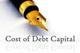# Cost of Debt

By Research DeskCost of debt refers to the effective interest paid by a company on its debts. While cost of debt can be calculated in either on before or after tax basis, since interest expense is deductible, generally the after-tax cost is considered.

Cost of debt measure is useful for giving an idea about the overall interest rate being paid for the debt financing since a company uses a variety of bonds, term loans and working capital from banks, external commercial borrowings (ECBs), commercial paper and other forms of debt. As a thump rule, higher cost of debt involves higher risk.

To calculate cost of debt, you’ll need two pieces of information – effective interest rate and marginal tax rate.

Effective Interest rate = (Annual Interest / Average Total Debt) * 100

Annual interest is taken from the income statement (note: If you're looking at a quarterly income statement, multiply this figure by four in order to annualize the data).

Average Total Debt is taken from the liabilities side of the balance sheet. Average is computed from the opening and closing debt.

Marginal Tax Rate = 1 - (Profit After Tax/Profit before tax)

To calculate the after-tax cost of debt, simply subtract the company's marginal tax rate from one and then multiply the result by the effective tax rate.

Cost of Debt = Effective Interest Rate * (1 - Marginal Tax Rate)

To illustrate this with an example, let us take the extract of financials of Dilip Buildcon for FY 2018:

Annual Interest = Rs. 464 crore

Total Debt = Rs. 2,770 crore

Profit Before Tax (PBT) = Rs. 664 crore

Profit After Tax (PAT) = Rs. 620 crore

Therefore,

Effective Interest Rate = (464/2770)*100 = 16.75%

Marginal Tax Rate = 1- (620/664) = 6.63%

Cost of Debt = 16.75% * (1 - 0.0663) = 15.64%